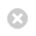Close

UCL Earth Sciences

Home# GEOL0060 Numerical Modelling of Mantle Dynamics for Earth and Planetary Sciences

The goal of this course is for students to learn how to program numerical applications from scratch. By the end of the course, students should be able to write state-of-the-art MATLAB codes that solve systems of partial-differential equations relevant to Earth and Planetary Science applications using finite-difference method and marker-in-cell technique. Applications include the Poisson equation, buoyancy-driven variable viscosity flow, heat diffusion and advection, and state-of-the-art thermomechanical code programming. The emphasis will be on commonality, i.e., using a similar approach to solve different applications, and modularity, i.e., re-use of code in different programs.

Coordinator: Dr Maxim Ballmer

Module details
 Title Numerical Modelling of Mantle Dynamics for Earth and Planetary Sciences UG Code GEOL0060 Coordinator Dr Maxim Ballmer Other Contributors Term 1 Credit 15 Written Exam Coursework 50% 200 lines of documented MATLAB computer code50% Homework, which is usually started during practical (8/9 are graded) Pre-Requisites GEOL0058; GEOL0057 (desirable) Maths & Stats Content and Requirement Total Number of Hours of Student Work 188 hours Hours of Lectures/Seminars 10 hours Hours of Practicals/Problem Classes 10 hours Hours of Tutorials 10 hours Days of Fieldwork 0 Other None
Content

In this module, students will learn how to write computer code in order to solve partial differential equations that are useful for Earth Science and Planetary applications. Programming is done in MATLAB, using the finite-difference method and marker-in-cell technique. The course will emphasise a hands-on learning approach rather than extensive theory. The goal of this module is to write a thermomechanical model of mantle convection from scratch.

In subsequent lectures/practicals, a series of modular computer codes are developed. These modular numerical models build on top of each other, and are ultimately integrated towards the fully-coupled thermo-mechanical code.

In the beginning, the basics of MATLAB programming will be briefly re-iterated. Then, we will move on to solve the 1D and 2D Poisson equation numerically using MATLAB. In the next step, the Momentum and Continuity Equations will be solved in a staggered grid using a 2D finite-difference discretization. In this purely mechanical approach, different boundary conditions and rheologies will be applied and tested. In a purely thermal approach, we will then solve the 2D Heat Equation for constant and variable thermal conductivity, also using finite differences on a staggered grid in MATLAB. Finally, the mechanical and thermal codes will be coupled towards a fully thermo-mechanical code with buoyancy-driven flow. Thermal advection will be addressed by the marker-in-cell technique. Step-by-step, internal heating, temperature-/pressure-dependent viscosity, temperature-dependent density and temperature-dependent thermal conductivity will be added to the thermo-mechanical code. The final project will include further development of this code to a fully-coupled thermo-chemical model with density also depending on composition.

#### AIMS

The goal of this course is for students to learn how to program numerical applications from scratch. By the end of the course, students should be able to write state-of-the-art MATLAB codes that solve systems of partial-differential equations relevant to Earth and Planetary Science applications using finite-difference method and marker-in-cell technique. Applications include the Poisson equation, buoyancy-driven variable viscosity flow, heat diffusion and advection, and state-of-the-art thermomechanical code programming. The emphasis will be on commonality, i.e., using a similar approach to solve different applications, and modularity, i.e., re-use of code in different programs.

#### OUTCOMES:

After completing this course, you will be able to understand as well as write from scratch a state-of-the-art thermomechanical numerical model in MATLAB. This code will be able to solve relevant problems in the Earth and Planetary Sciences. For example, you will understand the basics of fluid dynamics in planetary interiors with a wide range of applications, e.g. heat conduction and mantle convection (for details, see module description). In achieving this goal, you will also become familiar in documenting computer code, modifying existing code, and even independently writing your own code from scratch. You will have advanced your practical MATLAB skills, and learned how to efficiently solve partial differential equations.